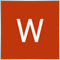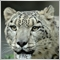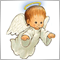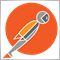# how to deduct array from another?81

hello

i searched every where how to deduct array elements from another but i didnt found such operate

lets say i have this

```int x_Array = {1, 2, 3, 4, 5};
int y_Array = {22, 2, 1, 5, 3, 65, 4};
int z_array[];
// i need to deduct x from y
//so result will be z_array={22, 65}```

thanx in advance for any help2220

am not an experiencedprogrammer but can I ask.
is x_Array represents the 6th candle and y_Array the 8th candle so then y_Array-x_Array_ is not z_Array

more like
```y_Array - x_Array = num;

//because Ithink z_Array has a set of defined characteristics that can not be redefined by the programmer more like the OrderSend()function```
I dont know but thats what I think882

```int x_Array = {1, 2, 3, 4, 5};
int y_Array = {22, 2, 1, 5, 3, 65, 4};
int z_array;
z_array=y_Array-y_Array;
// i need to deduct x from y
//so result will be z_array={22, 65}
z_array=y_Array;
z_array=y_Array;81

thank u GrumpyDuckMan for your help

but no its not like that

i just give a simple example, but x and y arrays are dynamic and also elements

here is the full code exactly

```int No_of_Positions = 3;
if(OrdersTotal() == No_of_Positions){
if(ArrayResize(History_Order_Ticket, OrdersHistoryTotal()) == OrdersHistoryTotal()){
for(int a = OrdersHistoryTotal() - 1; a >= 0; a--){
if(OrderSelect(a, SELECT_BY_POS, MODE_HISTORY)){
History_Order_Ticket[a] = OrderTicket();
}else{
History_Order_Ticket[a] = EMPTY;
}
}
}
ArrayCopy(History_Order_Ticket1, History_Order_Ticket, 0, 0, WHOLE_ARRAY);
//let us say History_Order_Ticket1[] will give 10 orders ticket

}else if(OrdersTotal() < No_of_Positions){
//this condition will take effect when 1 or 2 or any number of orders closed
if(ArrayResize(History_Order_Ticket2, OrdersHistoryTotal()) == OrdersHistoryTotal()){
for(int b = OrdersHistoryTotal() - 1; b >= 0; b--){
if(OrderSelect(b, SELECT_BY_POS, MODE_HISTORY)){
History_Order_Ticket2[b] = OrderTicket();
}else{
History_Order_Ticket2[b] = EMPTY;
}
}
}

//now History_Order_Ticket2[] will be bigger than History_Order_Ticket1[] with x of elements
//so i need to z_array[] = History_Order_Ticket2[] - History_Order_Ticket1[]

//i tried to use file open and delete but it not working well, i dont know where is the mistake
for(int c = 0; c < ArraySize(History_Order_Ticket2); c++){
string HOT = IntegerToString(History_Order_Ticket2[c]);
filehandle = FileOpen(HOT, FILE_WRITE|FILE_CSV);
FileClose(filehandle);
}
for(int d = 0; d < ArraySize(History_Order_Ticket1); d++){
FileDelete(IntegerToString(History_Order_Ticket1[d])))
}
```

Jefferson Metha thank you but what i want is not belong to a candle its simply

i need to get all closed orders ticket numbers not only the last order

i wish i got any help, more than one month searching but nothing882

Hello friend,

Sorry I failed mind reading at university. The two code are totally different. Ask the right questions and you might get the answers your after.81

GrumpyDuckMan:

Hello friend,

Sorry I failed mind reading at university. The two code are totally different. Ask the right questions and you might get the answers your after.

forgive me im so bad in english

i cant express the right questions :)3015

Wessam Nabil:

hello

i searched every where how to deduct array elements from another but i didnt found such operate

lets say i have this

thanx in advance for any help

```   int x_Array = {1, 2, 3, 4, 5};
int y_Array = {22, 2, 1, 5, 3, 65, 4};
int z_Array[];
int x_Size=ArraySize(x_Array),y_Size=ArraySize(y_Array);
bool found;
for(int i=0;i<y_Size;i++)
{
found=false;
for(int j=0;j<x_Size && !found;j++) found=y_Array[i]==x_Array[j];
if(!found)
{
int z_Size=ArraySize(z_Array);
ArrayResize(z_Array,z_Size+1);
z_Array[z_Size]=y_Array[i];
}
}
for(int i=0;i<ArraySize(z_Array);i++) printf("z_Array[%d]=%d",i,z_Array[i]);```81

Petr Nosek:

Petr Nosek

you r my hero, thats it

it works like a charm

thank you so much2392

The best way to manage this behavior is by using sets. If your data is only a few elements in size then it's no big deal to use nested loops and dynamically resizing arrays, however, if you're working with a larger collection then you'd want to get the difference using hashed sets. The MQL5 generic library also works with MQL4 so you can just copy to the MQL4/Include directory and use it. Example:

```#include <generic/hashset.mqh>

void OnStart()
{
int x_array[] = {1, 2, 3, 4, 5};
int y_array[] = {22, 2, 1, 5, 3, 65, 4};
int z_array[];
CHashSet<int> x_set(x_array), y_set(y_array);
y_set.ExceptWith(&x_set);
y_set.CopyTo(z_array);
for (int i=0; i<ArraySize(z_array); i++)
Print(z_array[i]);
}```